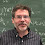## Tuesday, June 21, 2016

### When does n divide a_n? The answer, though you already know it. The Point, though its not as strong as I wanted. Oh well.

In my last post When does n divide a_n? I gave a sequence:

a(1)=0

a(2)=2

a(3)=3

for all n ≥ 4 a(n) = a(n-2) + a(n-3)

and I noted that for 2 ≤ n ≤ 23 it looked like

n divides a(n) iff n is prime

and challenged my readers to prove it or disprove it.

I thought it would be hard to find the first counterexample and hence I would make the point:

Just because a pattern holds for the first 271440  numbers does not mean that it always holds!

However, several readers found that 5212=271441 is a counterexample. In fact they found it rather quickly. I thought it would take longer since this blog (also my inspiration) by Colin Wright seemed to indicate that fancy data structures and algorithms tricks are needed. So I emailed Colin about this and it turns out he originally wrote the program many years ago. I quote his email:

---------------------------
> I posted on Perrin Primes (without calling them that) and people seemed to
> find the counterexample, 561^2, easily.

Yes.  These days the machines are faster, and languages with arbitrary
precision arithmetic are common-place.  When I first came across this
problem, if you wanted arithmetic on more than 32 bits you had to write
the arbitrary precision package yourself.  This was pre-WWW,
although not pre-internet.  Quite.

> So I wondered why your code needed those optimizations.

Even now, it's easy to find the first few counter-examples, but it rapidly
gets out-of-hand.  The sequence grows exponentially, and very soon you are
dealing with numbers that have thousands of digits. Again, not that bad now,
but impossible when I first did it, and even now, to find anything beyond the
first 20 or 30 counter-examples takes a very long time.

So ask people how to find the first 1000 counter-examples,
and what they notice about them all.
-----------------------------------------

back to my post:

It is known that if n is prime then n divides a(n). (ADDED LATER: see here for a proof) The converse is false. Composites n such  that n divides a(n) are called Perrin Pseudoprimes. The following questions seem open, interesting, and rather difficult:

1) Why is the first Perrin Pseudoprime so large?

2) Are there other simple recurrences b(n) where n divides b(n) iff n is prime LOOKS true for a while?

1.Could you link to a proof that if n is prime then n divides a(n)?

1.I've added a pointer to a proof in the post.

2.You seem to be claiming that you need to compute a(n) first and then check divisibility. This is, of course, not so. Any linear recurrence can be computed efficiently mod k through a standard trick involving matrices discussed in my book, Algorithmic Number Theory, and so it is trivial to look for counterexamples up to millions, with no need for extended precision integer arithmetic.

1.The point of the series of articles is to take the interested reader through the process of doing the obvious and naive through to the more complex and less obvious ideas. As you say, matrices can be used quickly and efficiently, working modulo the number we're interested in, to compute the result for a single number extremely quickly. We can trivially test numbers in the millions.

But the point is to go beyond millions. Each case is trivial to test, but testing all of them becomes impractical, hence needing the sieving techniques, and some way to avoid testing Every. Single. Number. All. The. Time.

3.4.